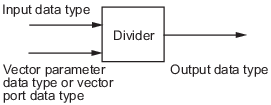# Array-Vector Divide

Divide array by vector along specified dimension

## Library

Math Functions / Matrices and Linear Algebra / Matrix Operations

`dspmtrx3`

•## Description

The Array-Vector Divide block divides the values in the specified dimension of the N-dimensional input array A by the values in the input vector V.

The length of the input V must be the same as the length of the specified dimension of A. The Array-Vector Divide block divides each element of V by the corresponding element along that dimension of A.

Consider a 3-dimensional M-by-N-by-P input array A(i,j,k) and an N-by-1 input vector V. When the Divide along dimension parameter is set to `2`, the output of the block Y(i,j,k) is

`$Y\left(i,j,k\right)=\frac{A\left(i,j,k\right)}{V\left(j\right)}$`

where

`$\begin{array}{l}1\le i\le M\\ 1\le j\le N\\ 1\le k\le P\end{array}$`

The output of the Array-Vector Divide block is the same size as the input array, A. This block accepts real and complex floating-point and fixed-point input arrays, and real floating-point and fixed-point input vectors.

### Fixed-Point Data Types

The following diagram shows the data types used within the Array-Vector Divide block for fixed-point signals.When you specify the vector V on the Main pane of the block mask, you must specify the data type and scaling properties of its elements in the Vector (V) parameter on the Data Types tab. When the vector comes in through the block port, its elements inherit their data type and scaling from the driving block.

You can set the vector and output data types in the block dialog.

## Parameters

Main Tab

Divide along dimension

Specify the dimension along which to divide the input array A by the elements of vector V.

Vector (V) source

Specify the source of the vector, V. The vector can come from the `Input port` or from a ```Dialog parameter```.

Vector (V)

Specify the vector, V. This parameter is visible only when you select `Dialog parameter` for the Vector (V) source parameter.

Data Types Tab

Note

Floating-point inheritance takes precedence over the data type settings defined on this pane. When inputs are floating point, the block ignores these settings, and all internal data types are floating point.

Rounding mode

Specify the rounding mode for fixed-point operations as one of the following:

• `Floor`

• `Ceiling`

• `Convergent`

• `Nearest`

• `Round`

• `Simplest`

• `Zero`

For more details, see rounding mode.

Saturate on integer overflow

When you select this parameter, the block saturates the result of its fixed-point operation. When you clear this parameter, the block wraps the result of its fixed-point operation. For details on `saturate` and `wrap`, see overflow mode for fixed-point operations.

Vector (V)

Use this parameter to specify the word and fraction lengths for the elements of the vector, V. You can set this parameter to:

• A rule that inherits a data type, for example, ```Inherit: Same word length as input```

• An expression that evaluates to a valid data type, for example, `fixdt(1,16,0)`

Click the buttonto display the Data Type Assistant, which helps you set the Vector (V) data type parameter.

Note

The Vector (V) parameter on the Data Types pane is only visible when you select ```Dialog parameter``` for the Vector (V) source parameter on the Main pane of the block mask. When the vector comes in through the block's input port, the data type and scaling of its elements are inherited from the driving block.

Output

Specify the output data type. See Fixed-Point Data Types for illustrations depicting the use of the output data type in this block. You can set it to:

• A rule that inherits a data type, for example, ```Inherit: Same as first input```

• An expression that evaluates to a valid data type, for example, `fixdt(1,16,0)`

Click the buttonto display the Data Type Assistant, which helps you set the Output parameter.

Minimum

Specify the minimum value that the block should output. The default value is `[]` (unspecified). Simulink® uses this value to perform:

• Simulation range checking (see Specify Signal Ranges (Simulink))

• Automatic scaling of fixed-point data types

Maximum

Specify the maximum value that the block should output. The default value is `[]` (unspecified). Simulink uses this value to perform:

• Simulation range checking (see Specify Signal Ranges (Simulink))

• Automatic scaling of fixed-point data types

Lock data type settings against changes by the fixed-point tools

Select this parameter to prevent the fixed-point tools from overriding the data types you specify on the block mask.

## Supported Data Types

PortSupported Data Types

A

• Double-precision floating point

• Single-precision floating point

• Fixed point (signed only)

• 8-, 16-, and 32-bit signed integers

V

• Double-precision floating point

• Single-precision floating point

• Fixed point (signed only)

• 8-, 16-, and 32-bit signed integers

Output

• Double-precision floating point

• Single-precision floating point

• Fixed point (signed only)

• 8-, 16-, and 32-bit signed integers

## Version History

Introduced in R2007b